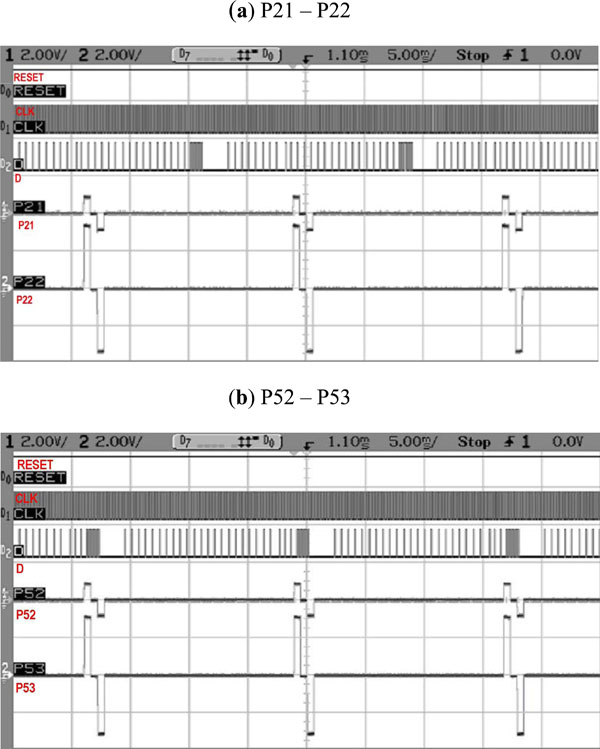Fig. (17) Variable data loaded (a) P21 = 0x20, P22 = 0x80 (b) P52 = 0x20, P53 = 0x80. Measured amplitudes are 0.8V and 3.2V respectively